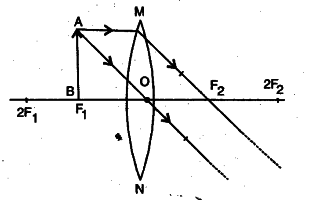# Define optical centre of a spherical lens

(a) Define optical centre of a spherical lens.
(b) You are given a convex lens of focal length 30 cm. Where would you place an object to get a real, inverted and highly enlarged image of the object? Draw a ray diagram showing the image formation.
© A concave lens has a focal length of 20 cm. At what distance from an object should an object be placed so that it forms an image at 15 cm away from the lens?

(a) It is the central point of the lens through which a ray of light passes without suffering any deviation.
(b) The object will be placed at F.© f = - 20 cm, u = ?, v = - 15 cm, h = ?

Using the expression

1/f = 1/v - 1/u , we have

1/-20 = 1/-15 - 1/u

u = 60 cm